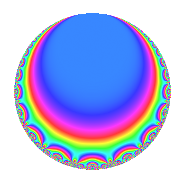# Properties

 Label 512.2.a.eLevel 512 Weight 2 Character orbit 512.a Self dual Yes Analytic conductor 4.088 Analytic rank 0 Dimension 2 CM No Inner twists 2

# Related objects

## Newspace parameters

 Level: $$N$$ = $$512 = 2^{9}$$ Weight: $$k$$ = $$2$$ Character orbit: $$[\chi]$$ = 512.a (trivial)

## Newform invariants

 Self dual: Yes Analytic conductor: $$4.08834058349$$ Analytic rank: $$0$$ Dimension: $$2$$ Coefficient field: $$\Q(\sqrt{2})$$ Coefficient ring: $$\Z[a_1, a_2, a_3]$$ Coefficient ring index: $$1$$ Fricke sign: $$-1$$ Sato-Tate group: $\mathrm{SU}(2)$

## $q$-expansion

Coefficients of the $$q$$-expansion are expressed in terms of $$\beta = \sqrt{2}$$. We also show the integral $$q$$-expansion of the trace form.

 $$f(q)$$ $$=$$ $$q$$ $$+ \beta q^{3}$$ $$+ 2 q^{5}$$ $$+ 2 \beta q^{7}$$ $$- q^{9}$$ $$+O(q^{10})$$ $$q$$ $$+ \beta q^{3}$$ $$+ 2 q^{5}$$ $$+ 2 \beta q^{7}$$ $$- q^{9}$$ $$-3 \beta q^{11}$$ $$+ 6 q^{13}$$ $$+ 2 \beta q^{15}$$ $$+ 3 \beta q^{19}$$ $$+ 4 q^{21}$$ $$-6 \beta q^{23}$$ $$- q^{25}$$ $$-4 \beta q^{27}$$ $$+ 2 q^{29}$$ $$-4 \beta q^{31}$$ $$-6 q^{33}$$ $$+ 4 \beta q^{35}$$ $$+ 6 q^{37}$$ $$+ 6 \beta q^{39}$$ $$+ 6 q^{41}$$ $$+ 3 \beta q^{43}$$ $$-2 q^{45}$$ $$+ q^{49}$$ $$-2 q^{53}$$ $$-6 \beta q^{55}$$ $$+ 6 q^{57}$$ $$- \beta q^{59}$$ $$+ 6 q^{61}$$ $$-2 \beta q^{63}$$ $$+ 12 q^{65}$$ $$-9 \beta q^{67}$$ $$-12 q^{69}$$ $$+ 6 \beta q^{71}$$ $$-12 q^{73}$$ $$- \beta q^{75}$$ $$-12 q^{77}$$ $$+ 4 \beta q^{79}$$ $$-5 q^{81}$$ $$-3 \beta q^{83}$$ $$+ 2 \beta q^{87}$$ $$-12 q^{89}$$ $$+ 12 \beta q^{91}$$ $$-8 q^{93}$$ $$+ 6 \beta q^{95}$$ $$-8 q^{97}$$ $$+ 3 \beta q^{99}$$ $$+O(q^{100})$$ $$\operatorname{Tr}(f)(q)$$ $$=$$ $$2q$$ $$\mathstrut +\mathstrut 4q^{5}$$ $$\mathstrut -\mathstrut 2q^{9}$$ $$\mathstrut +\mathstrut O(q^{10})$$ $$2q$$ $$\mathstrut +\mathstrut 4q^{5}$$ $$\mathstrut -\mathstrut 2q^{9}$$ $$\mathstrut +\mathstrut 12q^{13}$$ $$\mathstrut +\mathstrut 8q^{21}$$ $$\mathstrut -\mathstrut 2q^{25}$$ $$\mathstrut +\mathstrut 4q^{29}$$ $$\mathstrut -\mathstrut 12q^{33}$$ $$\mathstrut +\mathstrut 12q^{37}$$ $$\mathstrut +\mathstrut 12q^{41}$$ $$\mathstrut -\mathstrut 4q^{45}$$ $$\mathstrut +\mathstrut 2q^{49}$$ $$\mathstrut -\mathstrut 4q^{53}$$ $$\mathstrut +\mathstrut 12q^{57}$$ $$\mathstrut +\mathstrut 12q^{61}$$ $$\mathstrut +\mathstrut 24q^{65}$$ $$\mathstrut -\mathstrut 24q^{69}$$ $$\mathstrut -\mathstrut 24q^{73}$$ $$\mathstrut -\mathstrut 24q^{77}$$ $$\mathstrut -\mathstrut 10q^{81}$$ $$\mathstrut -\mathstrut 24q^{89}$$ $$\mathstrut -\mathstrut 16q^{93}$$ $$\mathstrut -\mathstrut 16q^{97}$$ $$\mathstrut +\mathstrut O(q^{100})$$

## Embeddings

For each embedding $$\iota_m$$ of the coefficient field, the values $$\iota_m(a_n)$$ are shown below.

For more information on an embedded modular form you can click on its label.

Label $$\iota_m(\nu)$$ $$a_{2}$$ $$a_{3}$$ $$a_{4}$$ $$a_{5}$$ $$a_{6}$$ $$a_{7}$$ $$a_{8}$$ $$a_{9}$$ $$a_{10}$$
1.1
 −1.41421 1.41421
0 −1.41421 0 2.00000 0 −2.82843 0 −1.00000 0
1.2 0 1.41421 0 2.00000 0 2.82843 0 −1.00000 0
 $$n$$: e.g. 2-40 or 990-1000 Significant digits: Format: Complex embeddings Normalized embeddings Satake parameters Satake angles

## Inner twists

Char. orbit Parity Mult. Self Twist Proved
1.a Even 1 trivial yes
4.b Odd 1 yes

## Atkin-Lehner signs

$$p$$ Sign
$$2$$ $$-1$$

## Hecke kernels

This newform can be constructed as the intersection of the kernels of the following linear operators acting on $$S_{2}^{\mathrm{new}}(\Gamma_0(512))$$:

 $$T_{3}^{2}$$ $$\mathstrut -\mathstrut 2$$ $$T_{5}$$ $$\mathstrut -\mathstrut 2$$ $$T_{7}^{2}$$ $$\mathstrut -\mathstrut 8$$